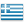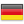# [LINQ vb.net] EXCEPT without removing duplicates

Hi. How do I remove all elements from one collection which exist in another collection without removing duplicates, using LINQ vb.net?

Please kindly modify the following code so that it works as intended.

``````Dim stringarray1 As String()
stringarray1 = {"hello","bye","bye"}

Dim stringarray2 As String()
stringarray2 = {"hello","hello","bye"}

Dim result1 As String()
result1 = stringarray1.Except(stringarray2)
Console.WriteLine(string.join(vbNewLine,result1))
' outputs nothing, but the desired output is "bye"

Dim result2 As String()
result2 = stringarray2.Except(stringarray1)
Console.WriteLine(string.join(vbNewLine,result2))
' outputs nothing, but the desired output is "hello"
``````
* LINQVisual Basic.NETLast Comment
louisfrHi,

You could use an extension method like the following:

``````Module ModuleExtensions
<Extension()>
Public Function ExceptWithDuplicates(aStringArray1 As String(),aStringArray2 As String()) As String()
Dim lStringArray = aStringArray1
.GroupBy(Function(x) x)
.Where(Function(x) x.Count > 1 OrElse Not aStringArray2.Contains(x.Key))
.Select(Function(x) x.Key)
.ToArray

Return lStringArray
End Function
End Module
``````

Essentially i am grouping the first array and figure out the count of each string. Then i am getting only those that either have a count of more than one or the ones that do not exist on the second array.

You may use this as in the example below:

``````   Dim stringarray1 As String()
stringarray1 = {"hello",  "bye", "bye"}

Dim stringarray2 As String()
stringarray2 = {"hello", "hello", "bye"}

Dim result1 As String()
result1 = stringarray1.ExceptWithDuplicates(stringarray2)
Console.WriteLine(String.join(Environment.NewLine,result1))
' outputs "bye"

Dim result2 As String()
result2 = stringarray2.ExceptWithDuplicates(stringarray1)
Console.WriteLine(String.join(Environment.NewLine,result2))
' outputs "hello"
``````louisfr

``````result1 = From s1 In stringarray1
Group Join s2 In stringarray2
On s1 Equals s2 Into Any
Where Not Any
Select s1
``````ste5anTHIS SOLUTION IS ONLY AVAILABLE TO MEMBERS.
View this solution by signing up for a free trial.
Members can start a 7-Day free trial and enjoy unlimited access to the platform.Thomas PAIK

Thanks to everyone for helping out.

@Ioannis Paraskevopoulos,
I am getting incorrect results for this set:

stringarray1 = {"hello","bye","bye"}
stringarray2 = {"hello","hello","hello","bye"}

result1 = {"bye"}, desired result = {"bye"}
result2 = {"hello"}, desired result = {"hello","hello"}

In otherwords, I would like to remove all elements from one collection which exist in another collection while preserving all duplicates.

Is it possible?

@louisfr,
GroupJoin is interesting.
Using Groupjoin, could you please provide an alternative LINQ vb.net code, similar to the style that Ioannis Paraskevopoulos implemented?
I am getting empty results (same as that of EXCEPT) on my system.
Thank you.

@ste5an,
I'm looking for a LINQ vb.net solution but it works fine.You may try something like this:

``````Module ModuleExtensions
<Extension()>
Public Function ExceptWithDuplicates(aStringArray1 As String(),aStringArray2 As String()) As String()
Dim lStringArray = aStringArray1 _
.GroupBy(Function(x) x) _
.SelectMany(Function(x)  _
x.Where(Function(y,i) _
i>0 OrElse Not aStringArray2.Contains(y))) _
.ToArray
Return lStringArray
End Function
End Module

``````

As a side note, why are you specifically looking for a LINQ solution? There are many cases when an alternate solution may be more elegant, more efficient and even more readable than LINQ. Do not get me wrong, I am a fan of LINQ, but if a solution works then it works.

That being said, if ste5an's answer works then you should select it.

Another comment is that it is a bit unclear what would you want to happen in the following scenario:

``````   Dim stringarray1 As String()
stringarray1 = {"hello","hello","bye","bye"}

Dim stringarray2 As String()
stringarray2 = {"hello","hello","hello","bye","bye"}
``````

ste5an's answer works by removing each element of the second array from the first array once, but if the second array has a repeating element, then it will be removed more than once.
``````results: "hello"
``````

My new solution suggested in this post only removes the first occurrence of the elements of the first array found in the second array.
``````results: "hello", "hello", "bye"
``````louisfr

Here is my solution without using Linq syntax:
``````result1 = stringarray1.GroupJoin(stringarray2,
Function(s1) s1,
Function(s2) s2,
Function(s1, sa2) New With {s1, sa2}).
Where(Function(x) Not x.sa2.Any).
Select(Function(x) x.s1)
``````
Linq syntax is clearer.Thomas PAIK

Thanks everyone for providing a second round of feedback.
I guess there is no clean LINQ solution to this problem.

@Ioannis Paraskevopoulos,
As you mentioned above, if there is an alternate solution that is more elegant, more efficient, and more readable than LINQ, you are more than welcome to provide the code. Thanks.

FYI, ste5an's solution gives the intended results:

stringarray1 = {"hello","hello","bye","bye"}
stringarray2 = {"hello","hello","hello","bye","bye"}

result1 = {""}, desired result = {""}
result2 = {"hello"}, desired result = {"hello"}

AND

stringarray1 = {"hello","hello","bye","bye"}
stringarray2 = {"hello","hello","hello","bye","bye","bye"}

result1 = {""}, desired result = {""}
result2 = {"hello","bye"}, desired result = {"hello","bye"}

@louisfr
It seems that I am not getting the intended results on my system.
Here are the results to both your codes:

stringarray1 = {"hello","bye","bye"}
stringarray2 = {"hello","hello","hello","bye"}

result1 = {""}, desired result = {"bye"}
result2 = {""}, desired result = {"hello","hello"}louisfr

I hadn't understood what you wanted exactly.
``````Dim result1 = stringarray1.
GroupBy(Function(s) s).
GroupJoin(stringarray2,
Function(s) s.Key,
Function(s) s,
Function(s1, s2) New With {
s1.Key,
.Count = s1.Count - s2.Count
}).
Where(Function(x) x.Count > 0).
SelectMany(Function(x) Enumerable.Repeat(x.Key, x.Count))
``````Visual Basic.NET

Visual Basic .NET (VB.NET) is an object-oriented programming language implemented on the .NET framework, but also supported on other platforms such as Mono and Silverlight. Microsoft launched VB.NET as the successor to the Visual Basic language. Though it is similar in syntax to Visual Basic pre-2002, it is not the same technology,

96K
Questions
--
Followers
--
Top Experts
Get a personalized solution from industry experts

TRUSTED BY